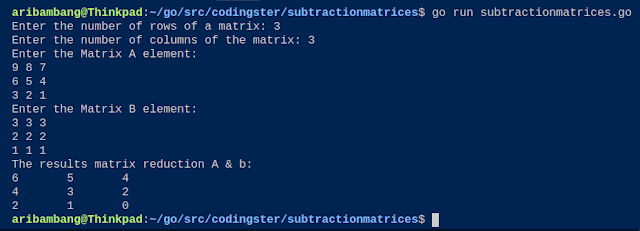-->

## Social Items

Go Program To Subtraction Two Matrices - in this post we will learn how to create a program to calculate the reduction of two matrices in Go programming language.

Matrix is a set of numbers arranged in rows (vertical) and columns (horizontal) can be referred to as a two-dimensional array. matrix reduction have terms that are the order of the matrix must be equal, or in other words, the two matrices must have the number of rows and columns are the same.

### Source Code :

``````
package main

import (
"fmt"
)

func main() {

var i, j, m, n int
var matriksA int
var matriksB int

fmt.Print("Enter the number of rows of a matrix: ")
fmt.Scanln(&m)
fmt.Print("Enter the number of columns of the matrix: ")
fmt.Scanln(&n)

fmt.Println("Enter the Matrix A element: ")
for i = 0; i < m; i++ {
for j = 0; j < n; j++ {
fmt.Scan(&matriksA[i][j])
}
}

fmt.Println("Enter the Matrix B element: ")
for i = 0; i < m; i++ {
for j = 0; j < n; j++ {
fmt.Scan(&matriksB[i][j])
}
}

fmt.Println("The results matrix reduction A & b: ")
for i = 0; i < m; i++ {
for j = 0; j < n; j++ {
fmt.Print(matriksA[i][j]-matriksB[i][j], "\t")
}
fmt.Println()
}
}
``````

Save the source code with the name of subtractionmatrices.go, but adjust wrote with a file name that chills and don't forget the extension should .go

### Compile & Run :

Here's how to compile source code manually:
``````
\$ go build subtractionmatrices.go
\$ ./subtractionmatrices
``````
You can run without having to compile it:
``````
\$ go run subtractionmatrices.go
``````

### The Output of Program :The Output of Program Subtraction Two Matrices (Golang)

### Conclusion :

From the results of the matrix reduction program can be run without any error and display the results of the subtraction of matrices A and matrix B.

### Video :## Go Program To Subtraction Two Matrices (Golang)

Go Program To Subtraction Two Matrices - in this post we will learn how to create a program to calculate the reduction of two matrices in Go programming language.

Matrix is a set of numbers arranged in rows (vertical) and columns (horizontal) can be referred to as a two-dimensional array. matrix reduction have terms that are the order of the matrix must be equal, or in other words, the two matrices must have the number of rows and columns are the same.

### Source Code :

``````
package main

import (
"fmt"
)

func main() {

var i, j, m, n int
var matriksA int
var matriksB int

fmt.Print("Enter the number of rows of a matrix: ")
fmt.Scanln(&m)
fmt.Print("Enter the number of columns of the matrix: ")
fmt.Scanln(&n)

fmt.Println("Enter the Matrix A element: ")
for i = 0; i < m; i++ {
for j = 0; j < n; j++ {
fmt.Scan(&matriksA[i][j])
}
}

fmt.Println("Enter the Matrix B element: ")
for i = 0; i < m; i++ {
for j = 0; j < n; j++ {
fmt.Scan(&matriksB[i][j])
}
}

fmt.Println("The results matrix reduction A & b: ")
for i = 0; i < m; i++ {
for j = 0; j < n; j++ {
fmt.Print(matriksA[i][j]-matriksB[i][j], "\t")
}
fmt.Println()
}
}
``````

Save the source code with the name of subtractionmatrices.go, but adjust wrote with a file name that chills and don't forget the extension should .go

### Compile & Run :

Here's how to compile source code manually:
``````
\$ go build subtractionmatrices.go
\$ ./subtractionmatrices
``````
You can run without having to compile it:
``````
\$ go run subtractionmatrices.go
``````

### The Output of Program :The Output of Program Subtraction Two Matrices (Golang)

### Conclusion :

From the results of the matrix reduction program can be run without any error and display the results of the subtraction of matrices A and matrix B.